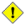## Name

= — Returns `TRUE` if the coordinates and coordinate order geometry/geography A are the same as the coordinates and coordinate order of geometry/geography B.

## Synopsis

`boolean =(` geometry A , geometry B `)`;

`boolean =(` geography A , geography B `)`;

## Description

The `=` operator returns `TRUE` if the coordinates and coordinate order geometry/geography A are the same as the coordinates and coordinate order of geometry/geography B. PostgreSQL uses the =, <, and > operators defined for geometries to perform internal orderings and comparison of geometries (ie. in a GROUP BY or ORDER BY clause).Only geometry/geography that are exactly equal in all respects, with the same coordinates, in the same order, are considered equal by this operator. For "spatial equality", that ignores things like coordinate order, and can detect features that cover the same spatial area with different representations, use ST_OrderingEquals or ST_EqualsThis operand will NOT make use of any indexes that may be available on the geometries. For an index assisted exact equality test, combine = with &&.

Changed: 2.4.0, in prior versions this was bounding box equality not a geometric equality. If you need bounding box equality, use ~= instead.This method supports Circular Strings and Curves.This function supports Polyhedral surfaces.

## Examples

```SELECT 'LINESTRING(0 0, 0 1, 1 0)'::geometry = 'LINESTRING(1 1, 0 0)'::geometry;
?column?
----------
f
(1 row)

SELECT ST_AsText(column1)
FROM ( VALUES
('LINESTRING(0 0, 1 1)'::geometry),
('LINESTRING(1 1, 0 0)'::geometry)) AS foo;
st_astext
---------------------
LINESTRING(0 0,1 1)
LINESTRING(1 1,0 0)
(2 rows)

-- Note: the GROUP BY uses the "=" to compare for geometry equivalency.
SELECT ST_AsText(column1)
FROM ( VALUES
('LINESTRING(0 0, 1 1)'::geometry),
('LINESTRING(1 1, 0 0)'::geometry)) AS foo
GROUP BY column1;
st_astext
---------------------
LINESTRING(0 0,1 1)
LINESTRING(1 1,0 0)
(2 rows)

-- In versions prior to 2.0, this used to return true --
SELECT ST_GeomFromText('POINT(1707296.37 4820536.77)') =
ST_GeomFromText('POINT(1707296.27 4820536.87)') As pt_intersect;

--pt_intersect --
f
```